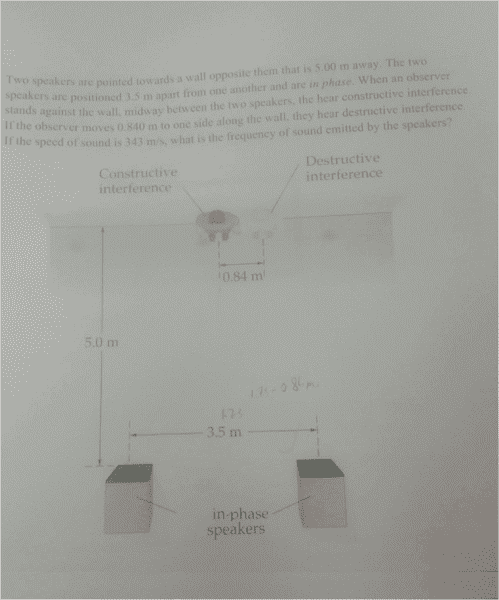# Can you please check this (destructive interference).

Sullo
If it is wrong can you please pinpoint where i am going wrong. Thank you.

1. Homework Statement

https://imgur.com/a/0STmmWt(uploaded picture because it has a diagram)

## Homework Equations

To sense destructive interference , the person must be one half wavelength (0.5λ) closer or farther from one speaker than the other.

## The Attempt at a Solution

Choosing distance from closer speaker (speaker on the right). So to sense destructive frequency he must be 0.5λ closer to the right speaker than the left.
Distance from right speaker before moving to the right (c^2) = a^2 + b^2
Distance from right speaker before moving to the right (c^2) = 1.75^2 + 5.0^2
Distance from right speaker before moving c = 5.30m

Distance from right speaker after moving to the right (c^2) = a^2 + b^2
Distance from right speaker after moving to the right c^2 = (1.75-0.84)^2 + (5.0)^2
Distance from right speaker after moving to the right c = 5.08m

Therefore; Distance from right speaker before moving - Distance from right speaker after moving
=5.30 - 5.08 = 0.22m

Therefore ; 0.22m = 0.5λ --> λ = 0.44m

v = fλ , f = v/λ = 343/0.44 = 779.5Hz

#### Attachments

Last edited by a moderator:

Mentor
The distance to the left speaker changes as well.

Sullo
The distance to the left speaker changes as well.
Can you please elaborate, why include both speakers in this?

Mentor
To sense destructive interference , the person must be one half wavelength (0.5λ) closer or farther from one speaker than the other.
The length difference between the left and right speaker has to be half the wavelength. What you calculated is something else - you didn't even consider the left speaker.

Sullo
The length difference between the left and right speaker has to be half the wavelength. What you calculated is something else - you didn't even consider the left speaker.

I'll need to go over this again tomorrow then

Sullo
The length difference between the left and right speaker has to be half the wavelength. What you calculated is something else - you didn't even consider the left speaker.
So do you mean distance from left speaker after moving + distance from right speaker after moving = 0.5λ ?

Mentor
The difference, not the sum.

Sullo
The difference, not the sum.
Oh ok. Thank you!

So is this correct?

Distance from left speaker after moving to the right (c^2) = a^2 + b^2
Distance from left speaker after moving to the right (c^2) = (1.75+0.84)^2 + 5.0^2
Distance from left speaker after moving to right c = 5.631

Distance from right speaker after moving to the right (c^2) = a^2 + b^2
Distance from right speaker after moving to the right c^2 = (1.75-0.84)^2 + (5.0)^2
Distance from right speaker after moving to the right c = 5.08m

Therefore; Distance from left speaker after moving - Distance from right speaker after moving
=5.631 - 5.08 = 0.551m

Therefore ; 0.551m = 0.5λ --> λ = 1.102m

v = fλ , f = v/λ = 343/1.102 = 311.26 Hz , 310hz (2 sig figs).

Mentor
That looks good.